# ILP Part 74 — Absolute value for real numbers

This is the seventieth fourth part of the ILP series. For your convenience you can find other parts in the table of contents in Part 1 – Boolean algebra

The original formula uses comparisons: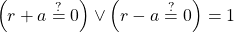where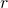should be an absolute value of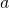. We know thatis at least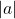and we use the formula above to enforce the equality. Effectively, we want to say thatis either equal toor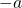We know that this is very dangerous as it implicitly requires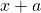to be at least. However, we don’t need to compare numbers precisely. What we need is a formula which will not allow forto be greater than. Let’s introduce this unreliable comparison: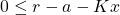if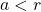thenmust be one. However, if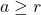then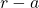is already non-positive socan be either zero or one.

However, important part for us is that ifthenwill be one. So now we can do two comparisons: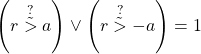If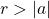then both comparisons would yield true which in turn would violate the constraint. Since we know thatis at leastthen there is no other way as to set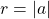. This works because solver can choose any result for unreliable comparisons.

This can be used for integer values as well. We can effectively calculate absolute value without any comparisons (which break the model for real numbers). Same goes for maximum and minimum, we can calculate these in a safe manner.